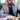January 14, 2021

# Problem Statement

Given a two-dimensional list of integers graph representing an undirected graph as an adjacency list, return a two-dimensional matrix M where

M[i][j] = 1 if there is a path between vertices i and j. M[i][j] = 0 otherwise. Constraints

1 ≤ n * m ≤ 100,000 where n and m are the number of rows and columns in graph

# Intuition

We use union-find data-structure with path compression to solve this.

For every edge perform union operation between the nodes

To see if nodes are connected perform find operation

# Implementation

We implement a unionfind class with path compression. For every edge (u,v) in the graph do uf.union(u, v)

To generate the matrix iterate over the whole matrix with pointers i and j and check uf.connected(i, j)

# Time Complexity

O(n^2) considering amortized constant time for union and find operation

# Space Complexity

O(n) is the additional space required for union find datastructure

# Code

``````class UnionFind:
def __init__(self, N):
self._parents = [i for i in range(N)]
self._n = N

def find(self, p: int):
root = p
while root != self._parents[root]:
root = self._parents[root]
true_root = root
while p != true_root:
temp = self._parents[p]
self._parents[p] = true_root
p = temp
return root

def connected(self, p: int, q: int):
return 1 if self.find(p) == self.find(q) else 0

def union(self, p: int, q: int):
root_p, root_q = self.find(p), self.find(q)
if root_p == root_q:
return
root = p
while root != self._parents[root]:
root = self._parents[root]

self._parents[root] = root_q

class Solution:
def solve(self, graph):
N = len(graph)
uf = UnionFind(N)
matrix = [ * len(graph) for i in range(N)]
for i, nodes in enumerate(graph):
for node in nodes:
uf.union(i, node)
for i in range(N):
for j in range(N):
matrix[i][j] = uf.connected(i, j)

return matrix``````Written by Ganesh Iyer A software engineer building platforms for leveraging artificial intelligence in healthcare.